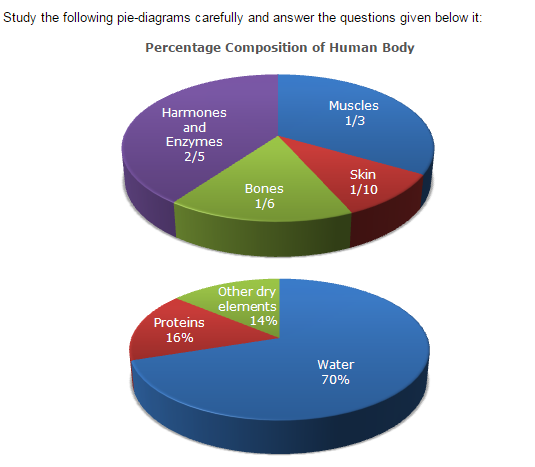Direction1.

What percent of the total weight of human body is equivalent to the weight of the proteins in skin in human body?

 A. 0.016 B. 1.6 C. 0.16 D. Data inadequate
Explanation: Let the body weight be x kg. Then, weight of skin protein in the body = [ 16% of ( 1 of x ) ] kg 10 = ( 16 x ) kg. 1000 Therefore Required percentage = [ ( 16x ) 1000 x 100 ] % = 1.6%. x

2.

What will be the quantity of water in the body of a person weighing 50 kg?

 A. 20 kg B. 35 kg C. 41 kg D. 42.5 kg
Explanation: Quantity of water in the body of a person weighing 50 kg = (70% of 50) kg = 35 kg.

3.

What is the ratio of the distribution of proteins in the muscles to that of the distribution of proteins in the bones?

 A. 1 : 18 B. 1 : 2 C. 2 : 1 D. 18 : 1
Explanation: Required ratio =16% of(1/3)/16% of(1/6) = 6/3=1/2

4.

To show the distribution of proteins and other dry elements in the human body, the arc of the circle should subtend at the centre an angle of:

 A. 54º B. 126º C. 108º D. 252º